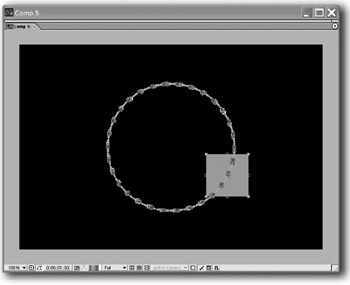# Useful Expressions I

### From Total Training:

Circle Fun by and Michael Natkin and Brian Maffitt

This generates perfectly circular motion centered around the original position of a layer. I recommend that you map the radius and cycle inputs to Expression Control sliders, and the phase input to an Expression Control angle.

Apply this expression to the position of the layer.

` radius = 75; // the radius of the circle cycle = 1; // number of seconds to complete a circle; higher value = slower if (cycle == 0) {cycle = 0.001;} //avoids a "divide by zero" error phase = 27; // initial angle in degrees from bottom reverse = 1; // 1 for ccw, -1 for cw X = Math.sin(reverse * degrees_to_radians(time * 360 / cycle + phase)); Y = Math.cos(degrees_to_radians(time * 360 / cycle + phase)); add(mul(radius, [X,Y]),position) `After Effects On the Spot[c] Time-Saving Tips and Shortcuts from the Pros
ISBN: 1578202396
EAN: N/A
Year: 2006
Pages: 447